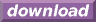#Calcute: freeware calculator

## Percent (pct, %)

A percent (or percentage) of a number is one hundredth of this number: the number in question is divided by 100. Calcute implements it using either the pct function or, equivalently, the % (percent) operator. For example pct(23) = 23% = 23/100.

```pct(23)
0.23
23%
0.23
```

Note that the percent operator (%) has higher precedence than the addition operator (+) in calculations. An operation like 23 + 10% does not add 10% of 23 (2.3) to 23, it adds 0.10 to 23. Adding 10% of 23 to 23 is done either explicitly by reusing 23 or by multiplying by 110% (100% + 10%):

```23 + 23*10%
25.3
23 * 110%
25.3
```